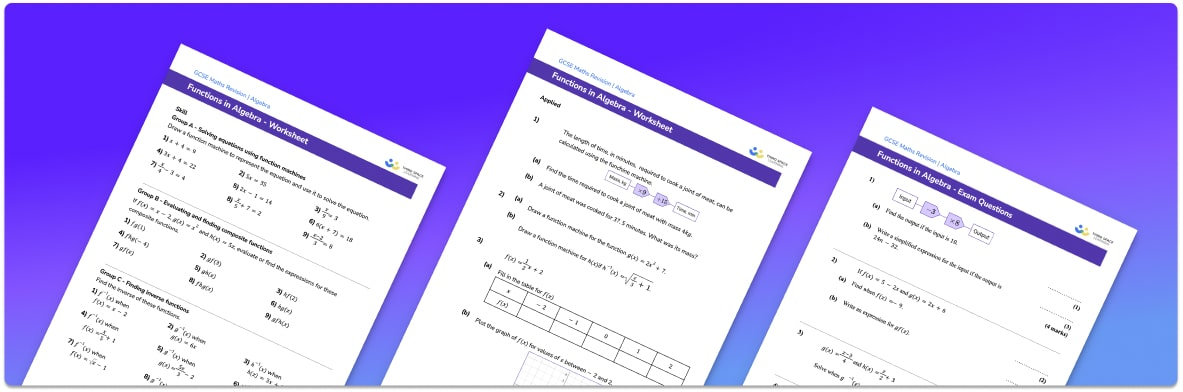# Functions Worksheet• Section 1 of the functions workbook contains 27 skills-based functions questions, in 3 groups to support differentiation
• Section 2 contains 4 applied functions questions with a mix of worded problems and deeper problem solving questions
• Section 3 contains 4 foundation and higher level GCSE workbook exam style questions for different types of equations
• Answers and a mark scheme for all functions questions are provided
• Questions follow variation theory with plenty of opportunities for students to work independently at their own level
• All questions created by fully qualified expert secondary maths teachers
• Suitable for GCSE maths revision for AQA, OCR and Edexcel exam boards

• This field is for validation purposes and should be left unchanged.

You can unsubscribe at any time (each email we send will contain an easy way to unsubscribe). To find out more about how we use your data, see our privacy policy.

### Functions at a glance

A function is a rule which represents the relationship between two variables. It has inputs and outputs. Functions can be represented in several ways including by using function machines (or number machines), by using algebra or by graphs. Functions expressed algebraically are often written as polynomials or sometimes as algebraic fractions.

In order to use functions it is important to have a strong understanding of the order of operations. Skills such as substitution into algebraic expressions and solving linear equations is also essential for functions.

There are different types of functions including linear functions, quadratic functions, reciprocal functions, exponential functions and trigonometric functions The graphs of linear functions are straight lines with a gradient and a y-intercept. The graphs of quadratic functions form a parabola. The graphs of reciprocal functions involve a rectangular hyperbola.

Functions can be combined by substituting one into another to form a composite function (or a composition of functions). Some functions have inverses which can be found by using skills similar to those involved in rearranging equations.

Looking forward, students can progress with more functions worksheets to additional algebra worksheets, for example a straight line graph or simultaneous equations worksheet.For more teaching and learning support on Algebra our GCSE maths lessons provide step by step support for all GCSE maths concepts.

## Do you have KS4 students who need more focused attention to succeed at GCSE?There will be students in your class who require individual attention to help them succeed in their maths GCSEs. In a class of 30, it’s not always easy to provide.

Help your students feel confident with exam-style questions and the strategies they’ll need to answer them correctly with our dedicated GCSE maths revision programme.

Lessons are selected to provide support where each student needs it most, and specially-trained GCSE maths tutors adapt the pitch and pace of each lesson. This ensures a personalised revision programme that raises grades and boosts confidence.

Find out more